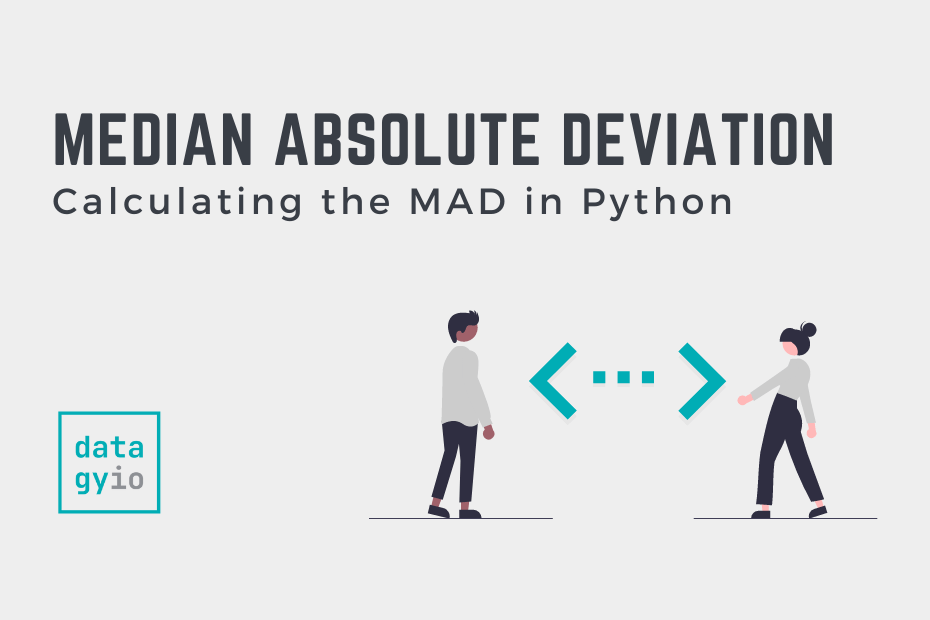# How to Calculate the Median Absolute Deviation in PythonThe median absolute deviation (MAD), is a robust statistic of variability that measures the spread of a dataset. In this tutorial, you’ll learn how to use Python to calculate the median absolute deviation.

By the end of this tutorial, you’ll have learned:

• What the Median Absolute Deviation is and how to interpret it
• How to use Pandas to calculate the Median Absolute Deviation
• How to use Scipy to Calculate the Median Absolute Deviation
• How to Use Numpy to Calculate the Median Absolute Deviation

## What is the Median Absolute Deviation?

The median absolute deviation is a measure of dispersion. This means that it is a measure that illustrates the spread of a dataset.

It is a particularly helpful measure because it is less affected by outliers than other measures such as variance. This is what makes the measure robust, meaning that it has good performance for drawing data.

The median absolute deviation (MAD) is defined by the following formula:

In this calculation, we first calculate the absolute difference between each value and the median of the observations. Then, we find the median value of that resulting array.

The median absolute deviation is a measure of dispersion that is incredibly resilient to outliers. In the following sections, you’ll learn how to use Python to calculate the median absolute deviation using a number of different libraries.

## How to Calculate the Median Absolute Deviation From Scratch in Python

Calculating the median absolute deviation from scratch using Python is quite simple! We can make use of the Statistics `median()` function and Python list comprehensions to make the process easy.

Let’s take a look at an example below:

``````# Calculating the median absolute deviation from scratch
from statistics import median

numbers = [86, 60, 95, 39, 49, 12, 56, 82, 92, 24, 33, 28, 46, 34, 100, 39, 100, 38, 50, 61, 39, 88, 5, 13, 64]
median_value = median(numbers)
median_absolute_deviation = median([abs(number-median_value) for number in numbers])
print(median_absolute_deviation)

# Returns: 16``````

Let’s break down what we’re doing here:

1. We loaded the `median()` function from statistics and initialized a list containing 25 values
2. We then calculated the median value using the `median()` function
3. We used a list comprehension to calculate the absolute difference between each item and the median value.
4. Finally, the median value of this resulting list was calculated.

In the following sections, you’ll learn how to calculate the median absolute deviation using scipy, Pandas, and Numpy.

## How to Calculate the Median Absolute Deviation in Scipy

The SciPy library comes with a function, `median_abs_deviation()`, which allows you to pass in an array of values to calculate the median absolute deviation.

Let’s see how we can easily replicate our above example to compute the median absolute deviation using Scipy.

``````# Using Scipy to Calculate the Median Absolute Deviation
from scipy.stats import median_abs_deviation

numbers = [86, 60, 95, 39, 49, 12, 56, 82, 92, 24, 33, 28, 46, 34, 100, 39, 100, 38, 50, 61, 39, 88, 5, 13, 64]
median_absolute_deviation = median_abs_deviation(numbers)
print(median_absolute_deviation)

# Returns: 16``````

We can see the same value is returned. Scipy also has a function, `median_absolute_deviation()`. Note, however, that this function was deprecated and should no longer be used.

## How to Calculate the Median Absolute Deviation in Pandas

There’ll be many times when you want to calculate the median absolute deviation for multiple columns in a tabular dataset. This is where Pandas comes into play.

While Pandas doesn’t have a dedicated function for calculating the median absolute deviation, we can use the apply method to accomplish this.

Let’s turn our list of numbers into a Pandas DataFrame column and calculate the median absolute deviation for it:

``````# Calculating the Median Abolute Deviation using Pandas
import pandas as pd
from scipy.stats import median_abs_deviation

numbers = [86, 60, 95, 39, 49, 12, 56, 82, 92, 24, 33, 28, 46, 34, 100, 39, 100, 38, 50, 61, 39, 88, 5, 13, 64]
df = pd.DataFrame(numbers, columns=['Numbers'])
print(df[['Numbers']].apply(median_abs_deviation))

# Returns:
# Numbers    16.0
# dtype: float64``````

We can see how easy it was to use the `median_abs_deviation()` function from Scipy to calculate the MAD for a column in a Pandas DataFrame.

## How to Calculate the Median Absolute Deviation in Numpy

In this final section, we’ll use pure Numpy code to calculate the median absolute deviation of a Numpy array. Because many Numpy functions allow us to work iteratively over arrays, we can simplify our earlier from-scratch example.

``````# Calculating the Median Abolute Deviation using Numpy
import numpy as np

numbers = np.array([86, 60, 95, 39, 49, 12, 56, 82, 92, 24, 33, 28, 46, 34, 100, 39, 100, 38, 50, 61, 39, 88, 5, 13, 64])

# Returns: 16.0``````

This code is a bit cleaner to read than the Python list comprehension example from earlier.

## Conclusion

In this tutorial, you learned how to calculate the median absolute deviation, MAD, using Python. You learned how to calculate it from scratch, as well as how to use Scipy, Numpy, and Pandas to calculate it in various ways.

The median absolute deviation represents a useful metric for the dispersion of a dataset’s observations. This is because it’s less influenced by outliers than other measures, such as the standard deviation.